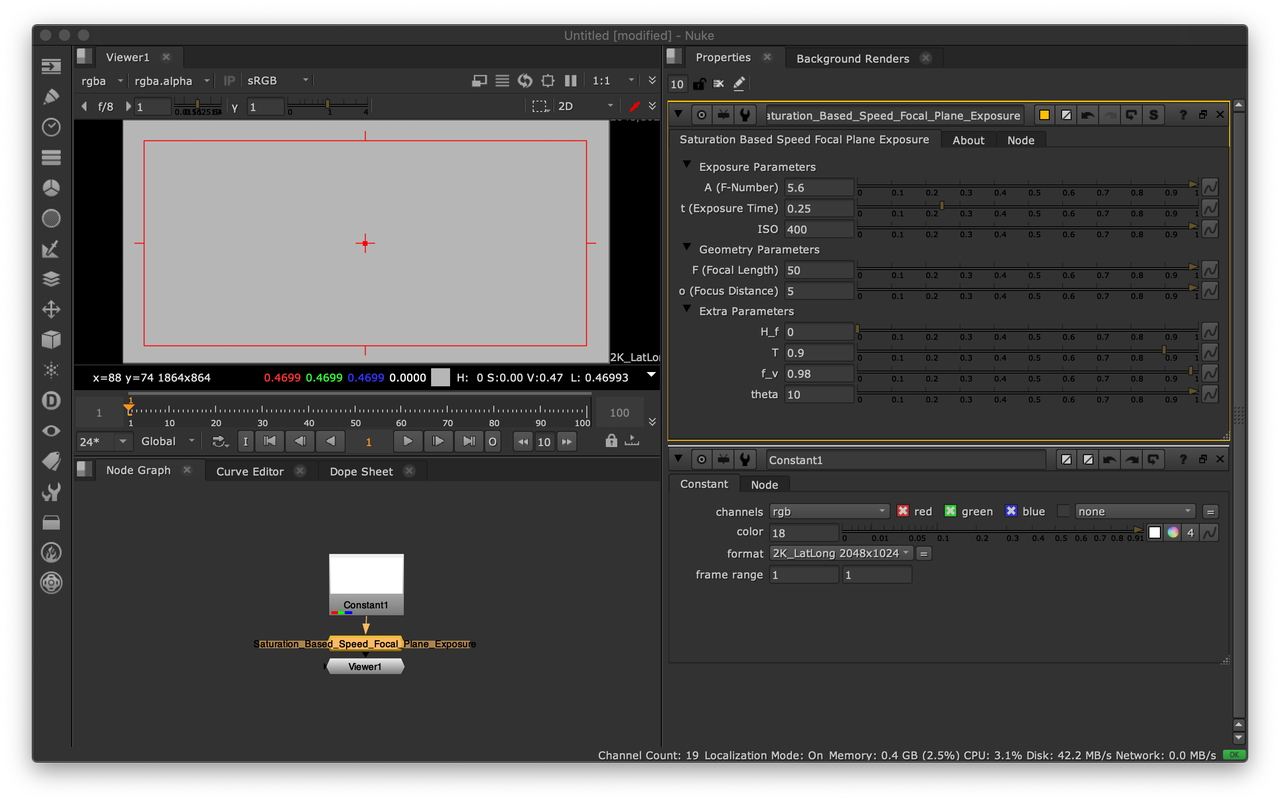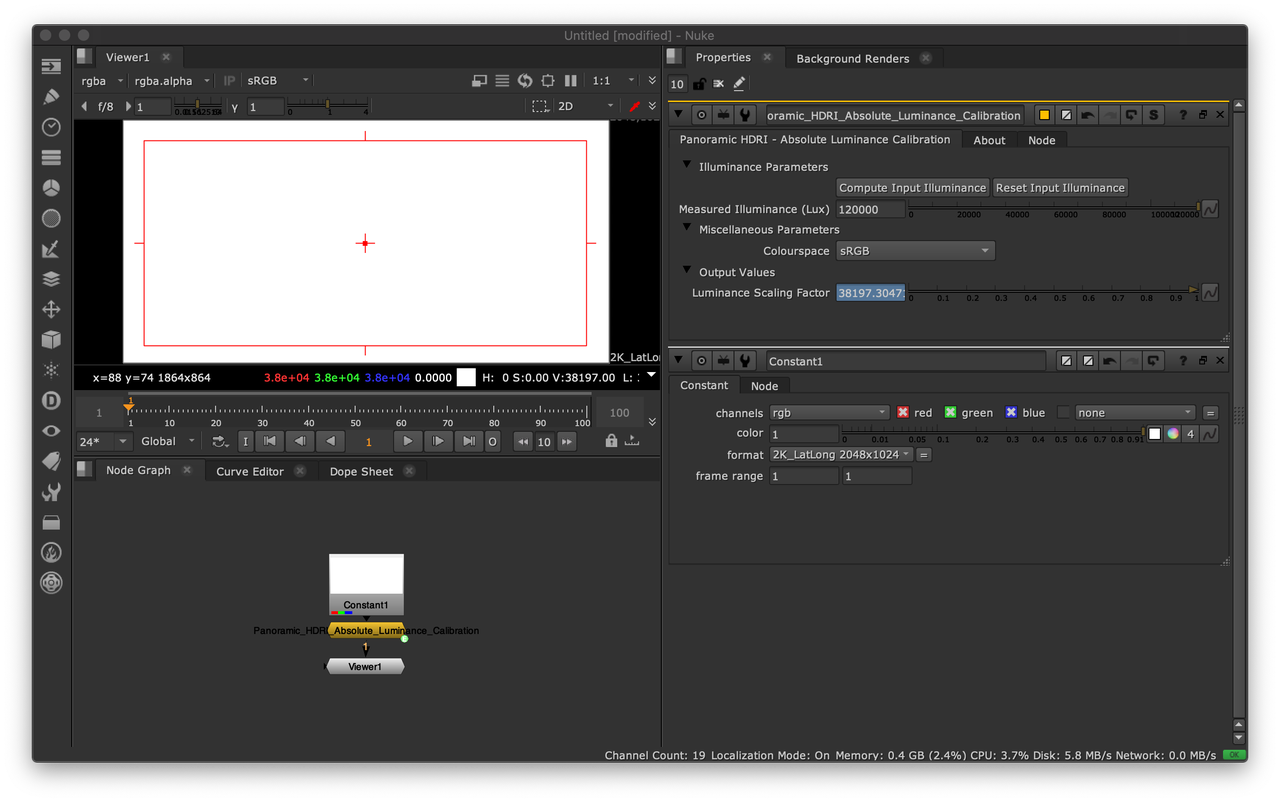# Absolute Luminance Calibration: Tying the Two Ends

Validation against ground truth data is an important step when implementing support for physical quantities in a realtime or offline renderer.

In this post, a simple but effective methodology to verify that the physical camera model behaves as expected will be presented.

## Digital Still Camera Exposure Model

Epic Games recently published a comprehensive blog post about auto-exposure handling in Unreal Engine 4.25. The part of most significance for this post is the mention of ISO Standard: 12232:2019.

Note

It is unfortunate that no reference is made to Moving Frostbite to Physically Based Rendering 3.0 by Lagarde and de Rousiers (2013). 7 years ago, they analytically derived the 1.2 scaling factor while citing the aforementioned ISO Standard.

Lagarde and de Rousiers (2013) describe a plausible Digital Still Camera (DSC) Exposure Model based on the Saturation-Based Speed (SBS) method.

The saturation based speed $S_{sat}$ of an electronic still picture camera is defined as:

$S_{sat}=\cfrac{78}{H_{sat}}$

where $H_{sat}$ is the minimum focal plane exposure, expressed in lux-seconds ($lx.s$), that produces the maximum valid (not clipped or bloomed) camera output signal. This provides $1/2$ "stop" of headroom (41% additional headroom) for specular highlights above the signal level that would be obtained from a theoretical 100% reflectance object in the scene, so that a theoretical 141% reflectance object in the scene would produce a focal plane exposure of $H_{sat}$.

The focal plane exposure $H$ in lux-seconds is given by the following equation:

$H=\cfrac{q L t F^2}{A^2 i^2} + H_f$

where

• $L$ is the scene luminance expressed in $cd/m^2$

• $A$ is the lens F-Number

• $t$ is the exposure time expressed in seconds

• $F$ is the lens focal length expressed in meters

• $I$ is the image distance expressed in meters

• $H_f$ is the focal plane flare exposure expressed in lux-seconds

• $q$ is the factor modeling the total lens vignetting and transmission attenuation:

$q=\cfrac{\pi T f_v \cos^4\theta}{4}$

with $T$ the transmission factor of the lens, $f_v$ the vignetting factor and $theta$ the angle of image point off axis. For a camera focused on infinity, $Hf<<H$, $T=9/10$, $\theta=10^{\circ}$, $\cos^4\theta=94/100$, and $fv=98/100$, $q$ is equal to 65/100.

The adjusted focal plane exposure $H_{SBS}$ is obtained by scaling the focal plane exposure $H$ according to the SBS method and, optionally, scaling by the ISO arithmetic speed $S$:

$H_{SBS}=H\cfrac{S}{100}\cfrac{100}{78}=H\cfrac{S}{78}$

Colour - HDRI implements the aforementioned model with Python:

>>> import colour_hdri
>>> colour_hdri.saturation_based_speed_focal_plane_exposure(18, 5.6, 0.25, 400)
0.46993364546604555


Colour - Nuke offers a Gizmo/Group implementation also available on Nukepedia.## Panoramic HDRI Calibration

With a plausible DSC Exposure Model implemented, calibrated ground truth data can (should) be imaged for verification purposes.

An Artist-Friendly Workflow for Panoramic HDRI by Lagarde, Lachambre and Jover (2016) describes a simple but effective process to calibrate a Panoramic HDRI for absolute luminance. The only requirement is to measuring the scene illuminance with a light meter during the HDRI capture.

The major advantage of this approach is that it is independent of the imaging device and thus does not require knowledge of its calibration constant $K$.

The multiplying factor $S_L$ used to convert the Panoramic HDRI relative luminance values to absolute luminance values is obtained as follows:

$S_L=\cfrac{E_{vm}}{E_{vi}}$

where $E_{vm}$ is the metered scene upper hemisphere illuminance in lux ($lx$) and $E_{vi}$ is the upper hemisphere illuminance of the Panoramic HDRI in lux, i.e. the upper hemisphere integral of the relative luminance values:

$\int_{\Omega}{L\ cos(\theta)\omega}$

For an equirectangular image, the solid angle $\omega$ of a pixel is given as follows:

$\omega=sin(\theta)\cfrac{2\pi}{w}\cfrac{\pi}{h}$

where $w$ and $h$ are the width and height of the image, respectively.

Colour - HDRI implements support for absolute luminance calibration with Python:

>>> import colour_hdri
>>> import numpy as np
>>> RGB = np.ones([2048, 1024, 3])
>>> colour_hdri.upper_hemisphere_illuminance_Lagarde2016(RGB)
>>> colour_hdri.absolute_luminance_calibration_Lagarde2016(RGB, 120000)[0, 0]
array([ 38215.85392444,  38215.85392444,  38215.85392444])
>>> colour_hdri.calibration.absolute_luminance.upper_hemisphere_illuminance_Lagarde2016(RGB)
3.1400580564615663

Note

Careful readers will have noticed that the last call to the colour_hdri.calibration.absolute_luminance.upper_hemisphere_illuminance_Lagarde2016 definition does not return $\pi$. This is induced by the numerical discretization to raster space, however, as image dimensions increase toward infinity, the computed value converges toward $\pi$, e.g. 3.1414009 and 3.1414968 for 16384x8192 and 32768x16384 sized images respectively.

Likewise, Colour - Nuke offers a Gizmo/Group implementation also available on Nukepedia.